Using Exponents and Polynomials Chapter Exam

Exam Instructions:

Choose your answers to the questions and click 'Next' to see the next set of questions. You can skip questions if you would like and come back to them later with the yellow "Go To First Skipped Question" button. When you have completed the practice exam, a green submit button will appear. Click it to see your results. Good luck!

Answered 0 of 30

Page 1

Question 4 4. Which of the following is equivalent to the following expression?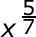Question 5 5. Which of the following would be true regarding the following exponential expression?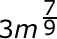Page 2

Question 6 6. Which of the following radical expressions is equal to this exponential expression?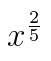Question 7 7. Simplify the expression using positive exponents.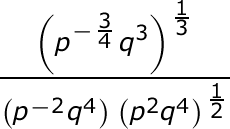Question 8 8. Simplify the expression using positive exponents.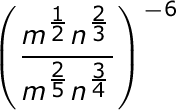Question 9 9. Simplify the expression using positive exponents.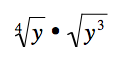Question 10 10. Simplify the following expression.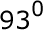Page 3

Question 11 11. Simplify using ONLY positive exponents.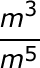Question 13 13. Divide using long division.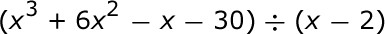Question 14 14. Divide using long division.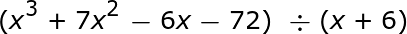Question 15 15. Divide using long division.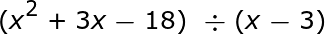Page 4

Question 16 16. Which of the following shows the graph of the equation below?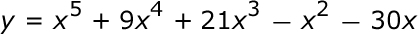Question 17 17. Which of the following shows the graph of the equation below?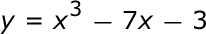Question 18 18. Which of the following shows the graph of the equation below?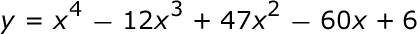Question 19 19. Simplify the expression below.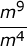Question 20 20. Which of the following is equivalent to the expression below?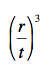Page 5

Question 22 22. Divide the following polynomials: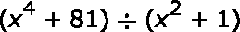Question 23 23. Divide the following polynomials: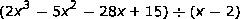Question 24 24. Divide the following polynomials: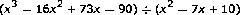Question 25 25. Simplify the expression below using positive exponents only.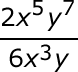Page 6

Question 26 26. Simplify the expression below using only positive exponents.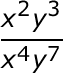Question 27 27. Simplify the following expression using positive exponents.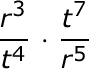Using Exponents and Polynomials Chapter Exam Instructions

Choose your answers to the questions and click 'Next' to see the next set of questions. You can skip questions if you would like and come back to them later with the yellow "Go To First Skipped Question" button. When you have completed the practice exam, a green submit button will appear. Click it to see your results. Good luck!

Support